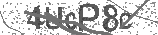﻿ Coulomb's Law (with example) | Safe Videos for Kids
Welcome

# Coulomb's Law (with example)

Thanks! Share it with your friends!

URL

You disliked this video. Thanks for the feedback!

Sorry, only registred users can create playlists.URLFind Related Videos  added
235 Views## Description

A simple, easy explanation of the intuition behind Coulomb's law and a worked example of an exam type question.

Hi! I'm Jade. I make fun physics videos that will make you smarter while making you smile :)

***SUBSCRIBE***

***Let's be friends***

***RELATED VIDEOS YOU WILL LOVE***
How to Calculate the Force Between THREE Charges https://www.youtube.com/watch?v=R8euHjXaIcE
What is an Electric Field? https://www.youtube.com/watch?v=LDJwqxpzA7A

____________________________________________________________________
1) Coulomb's law accurately measures the size of the force between two charges. It is based on two findings: the size of the electrostatic force is directly proportional to the product of the magnitude of the charges, and the electrostatic force is inversely proportional to the square of the distance separating the charges. When these two findings are translated into a mathematical equation it takes the form F=kq1q2/r^2, where F is the force, k is Coulomb's constant, q1 and q2 are the magnitudes of the charges, and r is the distance separating the charges. This is Coulomb's law.

2) The example worked through in this video tutorial is: Two charges of magnitude 1.8 x 10^-17 C are separated by a distance of 150 nm. What is the size of the electrostatic force experienced by each charge?Be the first to comment